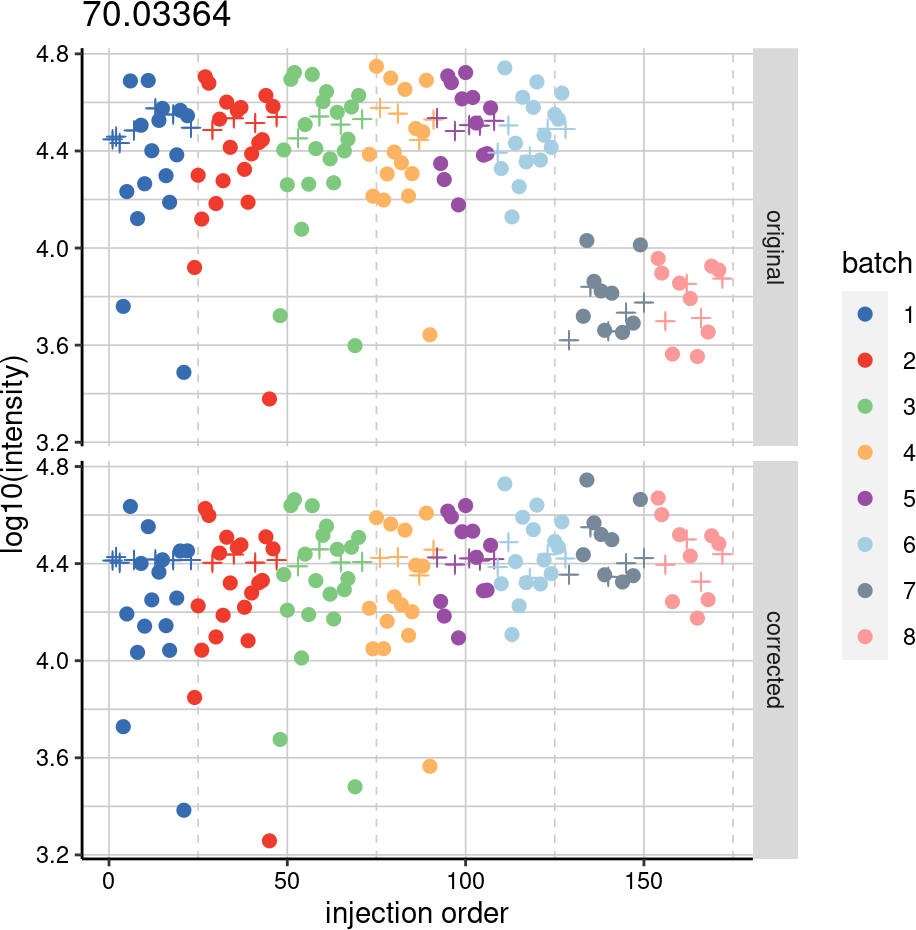# 1 Introduction

This vignette demonstrates how to apply Quality Control-Robust Spline Correction (QC-RSC) (Kirwan et al., 2013) algorithm for signal drift and batch effect correction within/across a multi-batch direct infusion mass spectrometry (DIMS) and liquid chromatography mass spectrometry (LCMS) datasets.

Please read “Signal drift and batch effect correction and mass spectral quality assessment” vignette to learn how to assess your dataset and details on algorithm itself.

# 2 Installation

You should have R version 4.0.0 or above and Rstudio installed to be able to run this notebook.

Execute following commands from the R terminal.

``````install.packages("gridExtra")

if (!requireNamespace("BiocManager", quietly = TRUE))
install.packages("BiocManager")
BiocManager::install("pmp")``````

Load the required libraries into the R environment

``````library(S4Vectors)
library(SummarizedExperiment)
library(pmp)
library(ggplot2)
library(reshape2)
library(gridExtra)``````

# 3 Dataset

In this tutorial we will be using a direct infusion mass spectrometry (DIMS) dataset consisting of 172 samples measured across 8 batches and is included in `pmp` package as `SummarizedExperiemnt` class object `MTBLS79`. More detailed description of the dataset is available from Kirwan et al. (2014), MTBLS79 and R man page.

``help ("MTBLS79")``
``````data("MTBLS79")

class <- MTBLS79\$Class
batch <- MTBLS79\$Batch
sample_order <- c(1:ncol(MTBLS79))

# Input data structure
MTBLS79
#> class: SummarizedExperiment
#> dim: 2488 172
#> assays(1): ''
#> rownames(2488): 70.03364 70.03375 ... 569.36369 572.36537
#> rowData names(0):
#> colnames(172): batch01_QC01 batch01_QC02 ... Batch08_C09 Batch08_QC39
#> colData names(4): Batch Sample_Rep Class Class2

class[1:10]
#>   "QC" "QC" "QC" "C"  "S"  "C"  "QC" "S"  "C"  "S"
batch[1:10]
#>   "1" "1" "1" "1" "1" "1" "1" "1" "1" "1"
sample_order[1:10]
#>    1  2  3  4  5  6  7  8  9 10``````

# 4 Missing values

Current implementation of `QCRSC` algorithm does support missing values in the input data object, but we would recommend to filter out features which were net reproducibly measured across quality control (QC) sample. In this example we will use 80% detection threshold.

``````data <- filter_peaks_by_fraction(df=MTBLS79, classes=class, method="QC",
qc_label="QC", min_frac=0.8)``````

# 5 Applying signal drift and batch effect correction

Function `QCRSC` should be used to apply signal batch correction.

Argument `df` should be `SummarizedExperiment` object or matrix-like R data structure with all `numeric()` values.

Argument `order` should be `numeric()` vector containing sample injection order during analytical measurement and should be the same length as number of sample in the input object.

Argument `batch` should be `numeric()` or `character()` vector containing values of sample batch identifier. If all samples were measured in 1 batch, then all values in the `batch` vector should be identical.

Values for `classes` should be character vector containing sample class labels. Class label for quality control sample has to be `QC`.

``````corrected_data <- QCRSC(df=data, order=sample_order, batch=batch,
classes=class, spar=0, minQC=4)
#> The number of NA and <= 0 values in peaksData before QC-RSC: 15330``````

# 6 Visual comparison of the results

Function ‘sbc_plot’ provides visual comparison of the data before and after correction. For example we can check output for features ‘1’, ‘5’, and ‘30’ in peak matrix.

``````plots <- sbc_plot (df=MTBLS79, corrected_df=corrected_data, classes=class,
batch=batch, output=NULL, indexes=c(1, 5, 30))
plots
#> []````````````#>
#> []``````